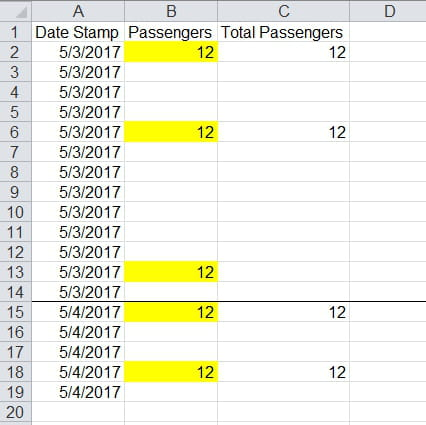# Need help with script or formula [Solved/Closed]

Posts
16
Registration date
Friday June 23, 2017
Status
Member
Last seen
November 20, 2017
-
josh07429 -
Column A shows "Date" while column B shows "Passenger #"
I want to Copy and paste the Passenger # to Column C "Total Passenger"

The problem is I only need to paste the the values from Column B to C twice on the same day/date.

as you can see in my photo, I have 3 "12" on 5/3/2017, but I only needed to paste them two of them at C. I ignored the 3rd "12" and moved on to the next day which is 5/4/2017 to do the same thing.

This is a little complex so I don't really know how to do this than use a complex script using macro.## 2 replies

Posts
2674
Registration date
Sunday September 12, 2010
Status
Moderator
Last seen
October 27, 2020
446
Hi Josh,

Give the following code a try:
```Sub RunMe()
Dim x, y As Integer

y = 2

NewDate:
x = 0
If Range("B" & y) <> vbNullString Then
Range("C" & y).Value = Range("B" & y).Value
x = 1
End If

Do
If Range("A" & y) = Range("A" & y - 1) And _
Range("B" & y) <> vbNullString And _
x < 2 Then
Range("C" & y).Value = Range("B" & y).Value
x = x + 1
End If

y = y + 1
If Range("A" & y) <> Range("A" & y - 1) Then GoTo NewDate

Loop Until Range("A" & y) = vbNullString
End Sub```

Hope you like it.

Best regards,
Trowa
THANK YOU!!

You're a life saver it worked :)

I only had a minor problem cause the dates have time but I just removed the time on the dates and your script works.

Thanks again.
Posts
16
Registration date
Friday June 23, 2017
Status
Member
Last seen
November 20, 2017

Hi, Is there a way to copy the highest value of the same day in column B to C? It seems that some of the numbers in B will sometimes have different numbers. I need to get the highest value and copy them to column C twice. Thanks in advance
Posts
2674
Registration date
Sunday September 12, 2010
Status
Moderator
Last seen
October 27, 2020
446
Hi Josh,

Quite the puzzle :)

See if the following code does the job:
```Sub RunMe()
Dim x, y, HighVal, SecHighVal, Rep As Integer
Dim mDate As Date

x = 2
y = 2
NextDate:

Do
mDate = Range("A" & x)
y = y + 1
Loop Until mDate <> Range("A" & y)

Range(Cells(x, "B"), Cells(y - 1, "B")).Select

HighVal = Application.WorksheetFunction.Max(Selection)

SecHighVal = Application.WorksheetFunction.Large(Selection, 2)

For Each cell In Selection
If cell.Value = HighVal And Rep < 2 Then
cell.Offset(0, 1).Value = cell.Value
Rep = Rep + 1
End If
Next cell

For Each cell In Selection
If cell.Value = SecHighVal And Rep < 2 Then
cell.Offset(0, 1).Value = cell.Value
Rep = Rep + 1
End If
Next cell

Rep = 0

If Range("A" & y).Value = vbNullString Then Exit Sub

x = y
GoTo NextDate

End Sub```

Let me know how the code performs!

Best regards,
Trowa

After changing some of the script it worked!!!

Thanks a lot :))))
Recommended

DON'T MISS